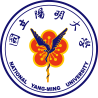# More1National Yang Ming University

## Explanation:

\frac{d[mRNAT7]}{dt}=\frac{ [ROSoxyR]^{nROSoxyR} }{ KdROSoxyR^{nROSoxyR}+[ROSoxyR]^{nROSoxyR} }\times{PoPStrxC}\times\frac{N}{V}\times{a}+\frac{ [T7]^{nT7} }{ KdT7^{nT7}+[T7]^{nY7} }\times{PoPST7}\times\frac{N}{V}-KdegmRNA\times[mRNAPDC]

PoPS represents the promoter strength which is measured by the rate of RNApolymerase binding to the starting site of DNA transcription; \frac{ [molecule]^{nmolecule} }{ Kdmolecule^{nmolecule}+[molecule]^{nmolecule} } represents the hill effect of transcription factors (either activators or repressors) to the promoter.

In this equation, the production rate of mRNAT7 is composed of both synthesizing rate and degrading rate. \frac{ [ROSoxyR]^{nROSoxyR} }{ KdROSoxyR^{nROSoxyR}+[ROSoxyR]^{nROSoxyR} }\times{PoPStrxC}\times\frac{N}{V}\times{a} represents how activator ROSoxyR influences promoter trxC on synthesizing mRNAT7 and how thresholds behind the promoter blocks the synthesis of mRNAT7 (the possibility of RNA polymerase to skip thresholds right after trxC promoter is shown in probability);\frac{ [T7]^{nT7} }{ KdT7^{nT7}+[T7]^{nY7} }\times{PoPST7｝\times\frac{N}{V}represents how T7 polymerase influences promoter T7 on synthesizing mRNAT7; -KdegmRNA\times[mRNAT7] represents the natural degrading rate of mRNAT7.